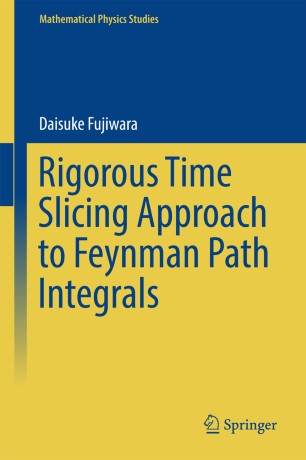# Rigorous Time Slicing Approach to Feynman Path Integrals

• Daisuke FujiwaraBook

Part of the Mathematical Physics Studies book series (MPST)

1. Front Matter
Pages i-ix
2. ### Convergence of Time Slicing Approximation of Feynman Path Integrals

1. Front Matter
Pages 1-1
2. Daisuke Fujiwara
Pages 3-19
3. Daisuke Fujiwara
Pages 21-38
4. Daisuke Fujiwara
Pages 39-77
5. Daisuke Fujiwara
Pages 79-95
6. Daisuke Fujiwara
Pages 97-135
7. Daisuke Fujiwara
Pages 137-186
3. ### Supplement–Some Results of Real Analysis

1. Front Matter
Pages 187-187
2. Daisuke Fujiwara
Pages 189-243
3. Daisuke Fujiwara
Pages 245-309
4. Daisuke Fujiwara
Pages 311-325
4. Back Matter
Pages 327-333

### Introduction

This book proves that Feynman's original definition of the path integral actually converges to the fundamental solution of the Schrödinger equation at least in the short term if the potential is differentiable sufficiently many times and its derivatives of order equal to or higher than two are bounded. The semi-classical asymptotic formula up to the second term of the fundamental solution is also proved by a method different from that of Birkhoff. A bound of the remainder term is also proved.
The Feynman path integral is a method of quantization using the Lagrangian function, whereas Schrödinger's quantization uses the Hamiltonian function. These two methods are believed to be equivalent. But equivalence is not fully proved mathematically, because, compared with Schrödinger's method, there is still much to be done concerning rigorous mathematical treatment of Feynman's method. Feynman himself defined a path integral as the limit of a sequence of integrals over finite-dimensional spaces which is obtained by dividing the time interval into small pieces. This method is called the time slicing approximation method or the time slicing method.
This book consists of two parts. Part I is the main part. The time slicing method is performed step by step in detail in Part I. The time interval is divided into small pieces. Corresponding to each division a finite-dimensional integral is constructed following Feynman's famous paper. This finite-dimensional integral is not absolutely convergent. Owing to the assumption of the potential, it is an oscillatory integral. The oscillatory integral techniques developed in the theory of partial differential equations are applied to it. It turns out that the finite-dimensional integral gives a finite definite value. The stationary phase method is applied to it. Basic properties of oscillatory integrals and the stationary phase method are explained in the book in detail.
Those finite-dimensional integrals form a sequence of approximation of the Feynman path integral when the division goes finer and finer. A careful discussion is required to prove the convergence of the approximate sequence as the length of each of the small subintervals tends to 0. For that purpose the book uses the stationary phase method of oscillatory integrals over a space of large dimension, of which the detailed proof is given in Part II of the book. By virtue of this method, the approximate sequence converges to the limit. This proves that the Feynman path integral converges. It turns out that the convergence occurs in a very strong topology. The fact that the limit is the fundamental solution of the Schrödinger equation is proved also by the stationary phase method. The semi-classical asymptotic formula naturally follows from the above discussion.
A prerequisite for readers of this book is standard knowledge of functional analysis. Mathematical techniques required here are explained and proved from scratch in Part II, which occupies a large part of the book, because they are considerably different from techniques usually used in treating the Schrödinger equation.

### Keywords

Feynman path integral Feynman propagator Fundamental solution Quantum mechanics Schroedinger equations Semiclassical techniques

#### Authors and affiliations

• Daisuke Fujiwara
• 1
1. 1.Department of MathematicsGakushuin UniversityTokyoJapan